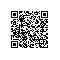# Oracle单表去重

create table A_TEST
(
id                 number,
name         varchar2(20),
remark varchar2(20)
);

insert into A_TEST (ID, NAME, REMARK)
values (1, 'a''ss');

insert into A_TEST (ID, NAME, REMARK)
values (2, 'b''xxx');

insert into A_TEST (ID, NAME, REMARK)
values (3, 'b''x');

insert into A_TEST (ID, NAME, REMARK)
values (4, 'b''asd');

insert into A_TEST (ID, NAME, REMARK)
values (5, 'c''axxx');

insert into A_TEST (ID, NAME, REMARK)
values (6, 'c''asdf');

select a.*
from A_TEST a, (select min(id) as id from A_TEST t group by name) b
where a.id = b.id;select *
from (select a.*, rownum row_num from A_TEST a) x
where x.row_num in (select min(rownum) from A_TEST t group by name)使用钉钉扫一扫加入圈子
+ 订阅